# First Missing Positive

Key takeaway: One of the best searching problems to learn step-by-step optimization using various approaches. In-place hashing solution is an intuitive problem-solving approach that uses the same input array to process values and generate correct output.

### Let’s understand the problem

Given an array that includes positive and negative numbers, write a program to find the smallest missing positive integer.

Example 1

Input: X[] = [2, -9, 5, 11, 1, -10, 7], Output: 3

Explanation: Consecutive positive integers 1 and 2 are present in the array. So the first missing positive is 3.

Example 2

Input: X[] = [2, 3, -2, 1], Output: 4

Explanation: Consecutive positive integers 1, 2, and 3 are present in the array. So the first missing positive is 4.

Example 3

Input: X[] = [4, 6, 5, 3, 8], Output: 1

Explanation: All values are positive, but the first positive integer 1 is missing in the input. So the output is 1.

### Discussed solution approaches

• Brute force approach  using two nested loops
• Using sorting and single scan
•  Using a Hash Table
• Using in-place hashing (an idea similar to counting sort)
• Using the idea of partition and In-place Hashing

### Brute force approach  using two nested loops

Solution Idea

The positive number starts from the value 1 on the number scale. If the first missing positive number is some number k, then all the positive numbers from 1 to k-1 will be present in the array. The critical question is: What would be the maximum possible value of missing positive if we have an array of size n? Think!

If the size of the array is n, then the maximum possible missing positive number would be n+1 i.e, the scenario when all numbers from 1 to n are present in the array. So basic approach would be to search all positive integers starting from 1 to n+1 linearly. If any positive integer is missing in the sequence, then we return that value as an output.

Solution Pseudocode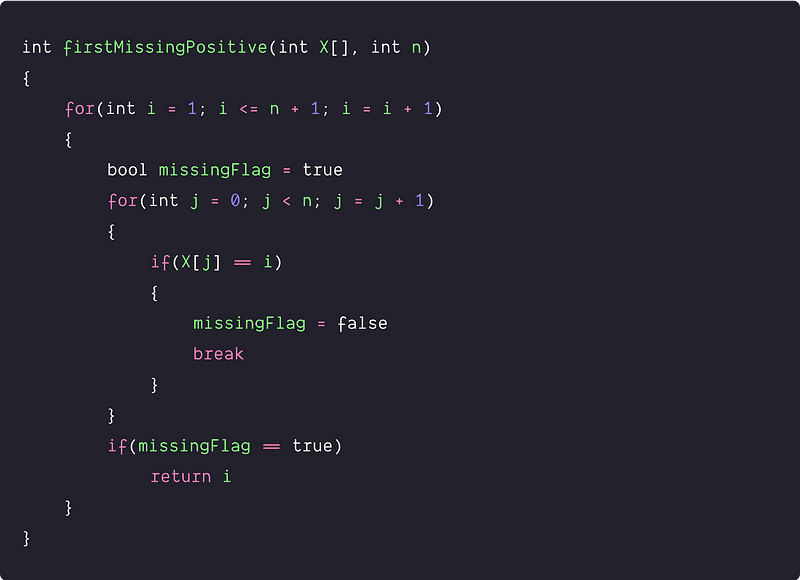Time and space complexity analysis

We are running two nested loops where both loops will run n times in the worst-case scenario. Time complexity in the worst-case = O(n²), Space Complexity = O(1)

### Using sorting and single scan

Solution Idea and Steps

If we sort the input array, then all the positive numbers get lined up in increasing order, and we can linearly search to find the first missing positive integer. We can use efficient sorting algorithms like heap sort, merge sort, or quicksort to sort the input array. Suppose we are using in-place O(nlogn) sorting algorithm heap sort.

1. We sort the array using heap sort.
2. We skip the negative numbers and zero by running a loop. Starting from the first index, when we find X[i] < 1, we increment i by 1. By the end of this loop, the index i will be present at the first positive number.
3. We run the next loop from j = i to n-1 to find the first missing positive number. Outside the loop, we also declare the variable missingPositive to track to the first missing positive. At the start, the missingPositive will be initialized with the smallest positive number i.e. 1.
4. We start comparing the missingPositive with X[i]. If(missingPositive == X[i]), we move to the next positive number in the sequence by incrementing missingPositive by 1.
5. We continue the above process until we find some value X[i] > missingPositive. In such a situation, the value stored in the variable missingPositive is the first missing positive. So we stop the loop and return missingPositive.

Solution Pseudocode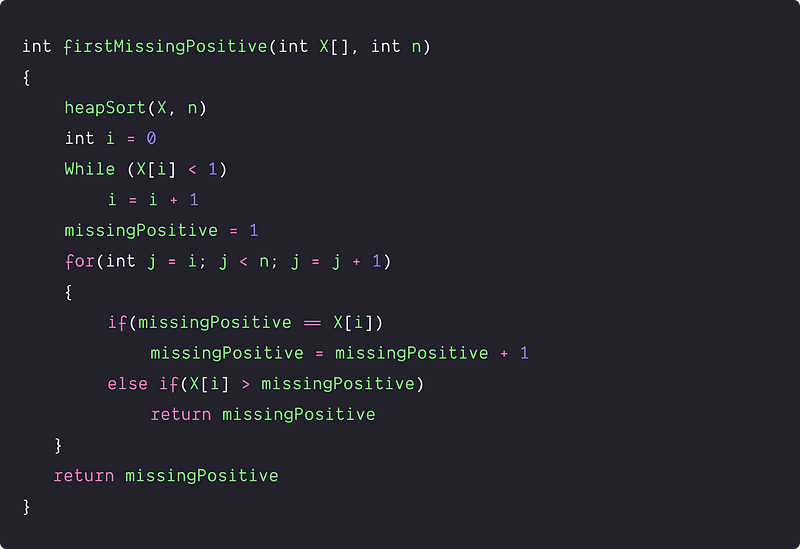Time and space complexity analysis

Time complexity = Time complexity of sorting + Linear scan for finding the first missing positive = O(nlogn) + O(n) = O(nlogn). Space complexity = O(1) (Think!)

### Using a hash table

Solution Idea

This is a searching problem where we need to look for positive integers starting from 1 to n + 1. To improve the time complexity further, we can use a hash table instead of a linear search to enhance the searching efficiency. The hash table performs searching and insertion efficiently in the O(1) average.

Solution Steps

• Create a hash table H of size n.
• Run a loop from i = 0 to n - 1 and insert all elements in the hash table H.
• Now run a loop from i = 1 to n + 1 to search all the possible positive numbers from 1 to n + 1 in the hash table H.
• If any positive integer i is not present in H, we return i. Otherwise, we move to the next iteration.

Solution Pseudocode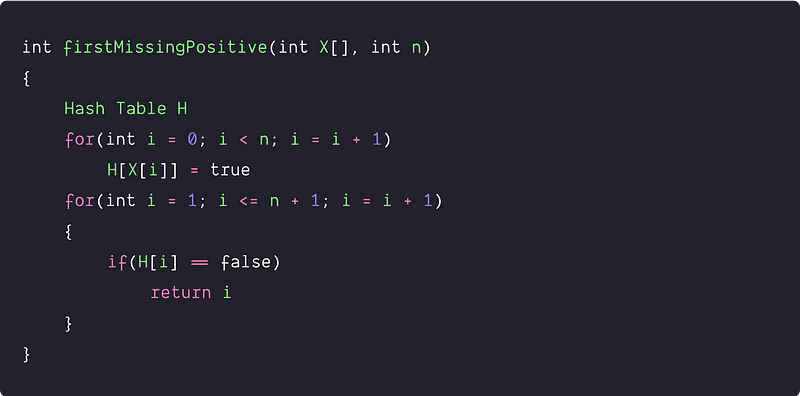Time and space complexity analysis

Time complexity = Inserting n elements in hash table + Searching n elements in hash table = O(n) + O(n) = O(n). Space complexity = O(n), for the hash table of size n.

### Using In-place hashing: The idea similar to counting sort

Solution Idea and Steps

Now the critical question is: can we solve this problem in place? Let’s think!

• We need to identify the first missing number in the sequence of 1 to n + 1. For this purpose, we can use the indexes of the same array to mark the presence of the numbers in the sequence and put each number in its correct place, i.e X [X[i] - 1] == X[i]. For example, we can place 1 at X, 2 at X, 3 at A, and so on.
• For placing the numbers in their right place, we scan the array X[] from the start and check: If the number is in the range of 1 to n and already present at index X[i] - 1 or not. If the first condition is true and the second is false, we need to place the number at index X[i] by swapping it with the number present at the index X[i] - 1. Otherwise, we ignore the number and move forward to the next index.
• We need to scan the modified array again to find the first index where X[i] != i+1. For some value of i, if X[i] != i+1, then we return that index. If we didn't find any such index, all numbers from 1 to n are present in the array, and we need to return the value n + 1 as the first missing positive.

The best part of this idea is: we are modifying the same array and storing the results to get the correct output.

Solution Pseudocode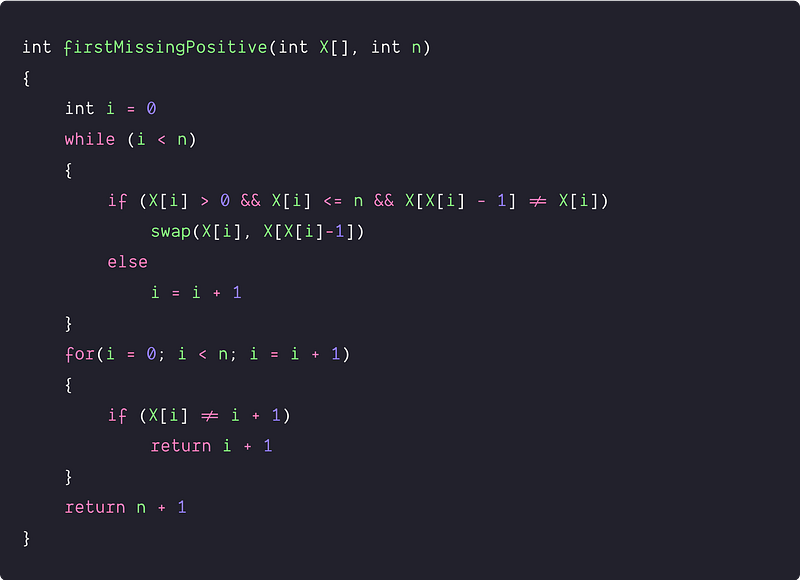Time and space complexity analysis

We visit each number and put it in its right place at most once. In another way, we are running two separate single loops n times. So time complexity in the worst case = time complexity of modifying the array + time complexity of searching first missing positive = O(n) + O(n) = O(n)

Space complexity = O(1), as we use the same input array to place the elements at their correct position. That's why such approaches are always a premium approach to problem-solving!

### Using partition and In-place hashing

Solution Idea and Steps

Here is an idea similar to the above approach, but here we first divide the array into two parts: the first part consists of positive numbers, and the second part consists of negative numbers. We are assuming the array can be modified.

• Using the partition algorithm similar to the quick sort, we partition the input array into a group of positive and negative numbers. After the partition, the positive number starts from index 0 and ends at index positiveEnd - 1.
• Now we traverse the array from the index 0 to positiveEnd - 1. If (X[i] > positiveEnd) then we do nothing. If not, we make the sign of the element at the index X[i] - 1 negative (Think!)
• Finally, we traverse the array once more from index 0 to positiveEnd -1. If we encounter a positive element at some index i, we output i + 1 as the first missing positive. However, if we do not encounter any positive element, integers 1 to positiveEnd occur in the array and return positiveEnd + 1 as an output. It can also be the case that all the numbers are non-positive, making positiveEnd = 0. The output positiveEnd + 1 = 1 remains correct.

We can better understand this approach via an example.

Initial Array: 1 -1 -5 -3 3 4 2 8

Step 1: Partition: 1 8 2 4 3 | -3 -5 -1, positiveEnd = 5

Step 2: Modifying the input array.

• 1 < positiveEnd, we change the sign of value at index 1 - 1 = 0

Array changes to: -1 8 2 4 3 | -3 -5 -1

• 8 > positiveEnd, we do nothing and move to the next element.
• 2 < positiveEnd, we change the sign of value at index 2 - 1 = 1

Array changes to: -1 -8 2 4 3 | -3 -5 -1

• 4 < positiveEnd, we change the sign of value at index 4 - 1 = 3

Array changes to: -1 -8 2 -4 3 | -3 -5 -1

• 3 < positiveEnd, we change the sign of value at index 3 - 1 = 2,

Array changes to: -1 -8 -2 -4 3 | -3 -5 -1

Step 3: Traversing from index 0 to positiveEnd, we find X = 3 > 0. The answer is 4 + 1 = 5

Why this approach is working perfectly? What is the critical reason behind it? We are leaving this intuition for the learners to think! Enjoy thinking!

Solution Pseudocode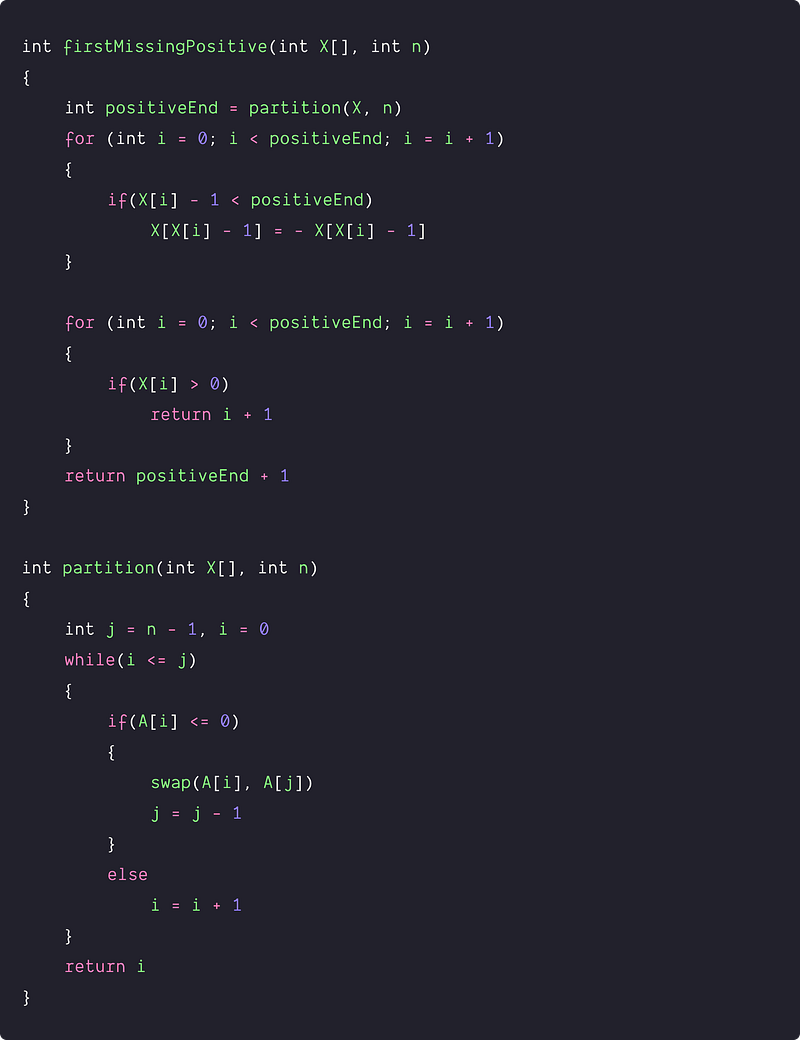Time and space complexity analysis

Time complexity = Time complexity of the partition + time complexity of modifying the input array + Linear scan to find the missing positive = O(n) + O(n) + O(n) = O(n)

Space complexity = O(1), we are solving the problem in place using the same input array!

### Critical ideas to think!

• Why are we not storing the occurrences of numbers greater than n?
• What if we need to find the smallest missing number from a given set of numbers?
• How do we solve when we need to find the largest missing negative number?
• Is it possible to output all the numbers missing in the array in the range [1,n]?
• In the 2nd approach, our array is sorted. so, can we think to optimize the search by applying the binary search algorithm?
• Can we solve the problem using some other approach?
• Will the 4th and 5th approaches work if the input array contains large numbers?
• In the 3rd approach, do we need to store all non-positive integers?
• What if all the elements that are present in the array are negative? Do the above algorithms cover this condition?

### Comparisons of time and space complexities

• Using two nested loops: Time = O(n^2), Space = O(1)
• Using sorting and single scan: Time = O(nlogn), Space = O(1)
•  Using a Hash Table: Time = O(n), Space = O(n)
• Using idea similar to counting sort: Time = O(n), Space = O(1)
• Using partition and In-place Hashing: Time = O(n), Space = O(1)

### Suggested coding problems to practice

• Find all numbers that disappeared in an array.
• The kth missing element in an unsorted array.
• Smallest prime number missing in an array.
• Find the missing integer in an array if the mean is given.
• Find the missing number in another array which is a shuffled copy.

Enjoy learning, Enjoy algorithms!

Get well-designed application and interview centirc content on ds-algorithms, machine learning, system design and oops. Content will be delivered weekly.